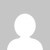# 17EC36 Engineering Electromagnetics VTU Notes

[wptelegram-join-channel]

## 17EC36 Engineering Electromagnetics VTU Notes

Download VU CBCS notes of 17EC36 Engineering Electromagnetics for 3rd-semester Electronics and Communications Engineering, VTU Belagavi.

### Module 1 -Coulomb’s Law, Electric Field Intensity and Flux density

Following are the contents of module 1 – Coulomb’s Law, Electric Field Intensity, and Flux density

Introduction to Coulomb’s Law, Electric Field Intensity and Flux density. Law of Coulomb, Electric field intensity,  charge distribution,  line charge, flux density.

### Module 2 – Gauss’s law and Divergence

Following are the contents of module 2 –

Introduction to Gauss’s law and Divergence and Energy, Potential and Conductors.
Definition of potential difference and potential field of a point charge, Current, Current density, and Continuity of current.

### Module 3 – Poisson’s and Laplace’s Equations

Following are the contents of module 3 – Poisson’s and Laplace’s Equations

Introduction to Poisson’s and Laplace’s Equations, Steady Magnetic Field.
Poisson’s and Laplace’s Equations derivation. Uniqueness theorem and  Laplace’s equation simple examples.
Biot-Savart, Ampere’s circuital, Curl and Stokes’ theorem

### Module 4 – Magnetic Forces and Materials

Following are the contents of module 4 – Magnetic Forces and Materials

Introduction to Magnetic Forces and Materials. Force on a moving charge, current elements, between differential current elements.

### Module 5 – Time-varying fields and Maxwell’s equations

Following are the contents of module 5 – Time-varying fields and Maxwell’s equations

Introduction, concepts, and basic principles of Time-varying fields and Maxwell’s equations and Uniform Plane Wave.

M-1, M-2, M-3, M4 and M-5

ECE VTU Question Papers

### 2018 Scheme VTU  Notes Electronics and Communications Engineering

If you like VTU CBCS notes, VTU end exam and model question papers, various study material, and for regular updates do like the Facebook page and YouTube channel for video tutorials.

### 2 thoughts on “17EC36 Engineering Electromagnetics VTU Notes”

1.Hllo sir,
1.